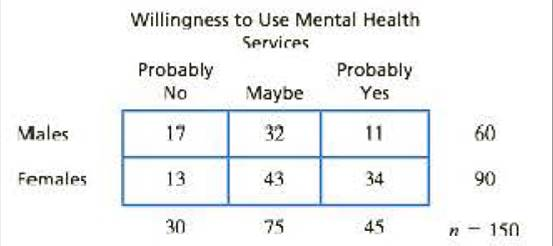Chapter 15, Problem 12PEssentials of Statistics for the B...

8th Edition
Frederick J Gravetter + 1 other
ISBN: 9781133956570

Solutions

Chapter
SectionEssentials of Statistics for the B...

8th Edition
Frederick J Gravetter + 1 other
ISBN: 9781133956570
Textbook Problem

Research has demonstrated strong gender differences in teenagers’ approaches to dealing with mental health issues (Chandra & Minkovitz. 2006). In a typical study, eighth-grade students are asked to report their willingness to use mental health services in the event they were experiencing emotional or other mental health problems. Typical data for a sample of n = 150 students are shown in the following table.a. Do the data show a significant relationship between gender and willingness to seek mental health assistance? Test with α = .05. b. Compute Cramér’s V to measure the size of the treatment effect.

a.

To determine

To check: Whether there is significant relationship between gender and willingness to seek mental health assistance for the given question.

Explanation

Given info:

A sample of 150 students was involved in a study based on “the willingness to use mental health services and gender”. Use α=0.05 to test the claim.

Calculations:

Step 1: Null Hypothesis and Alternate Hypothesis are:

H0: There is no significant relationship between gender and willingness to seek mental health assistance.

H1: There is a significant relationship between gender and willingness to seek mental health assistance.

Step 2: For the given sample, degrees of freedom equals:

df=(R1)(C1)  where R equals number of rows and C equals columns=(21)(31)=2

With α=0.05 and df=2 , the critical value (CV)  is obtained from the χ2table as

χ2=5.991

Step 3: χ2statistics is calculated as:

χ2=(fofe)2fe

The formula to calculate expected frequency is:

fe=fcfrnwhere fr is row frequency and fc is column frequency

Substitute n=200 in the above formula and compute respective values of expected frequencies:

For the category “males”, the expected frequencies are:

fe,prob.no=30×60150fe,yes=75×60150fe,prob.no=45×60150

Similarly, for the category “females”, the expected frequencies are:

fe,prob

b.

To determine

To Find: The value of Cramer’s V for the given question.

Still sussing out bartleby?

Check out a sample textbook solution.

See a sample solution

The Solution to Your Study Problems

Bartleby provides explanations to thousands of textbook problems written by our experts, many with advanced degrees!

Get Started

Find the value of the sum. 26. i=11004

Single Variable Calculus: Early Transcendentals, Volume I

Find f'(a). f(x) = x2

Calculus: Early Transcendentals

True or False: If converges and converges, then converges.

Study Guide for Stewart's Single Variable Calculus: Early Transcendentals, 8th

π does not exist

Study Guide for Stewart's Multivariable Calculus, 8th